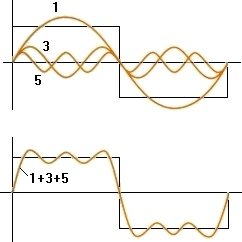# Fourier Analysis

Fourier series

A Fourier series is a way to represent a function as the sum of simple sine waves.
A simple trigonometric function consists of a smooth surface. If you continue to add these trigonometric functions, you can get a function with a different shape.
For example, let’s create a square function using a Fourier series.As shown in the figure above, you can get a shape similar to a rectangular function by adding three ordinary trigonometric functions. Here is a list of expressions.

$f(x)\, =\, sin(x)\, +\, \frac { 1 }{ 3 } sin(3x)\, +\, \frac { 1 }{ 5 } sin(5x)$

Now this is only a combination of three waves, so it is not yet a complete rectangle.However, if you do this infinitely, you get a clean function.

\begin{align} f(x)\, &=\, sin(x)\, +\, \frac { 1 }{ 3 } sin(3x)\, +\, \frac { 1 }{ 5 } sin(5x)\, +\,… \\ &=\, \sum _{ n=0 }^{ \infty }{ \frac { 1 }{ (2n+1) } sin((2n+1)x) } \end{align}

Application of Fourier series

Fourier series is often used to obtain a specific waveform in an electronic circuit.
For example, the Fourier series for squares and sawtooths is: